# Computer arithmetic

29 de Mar de 2015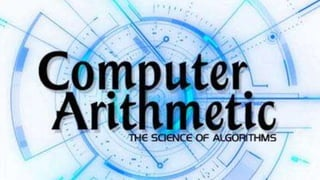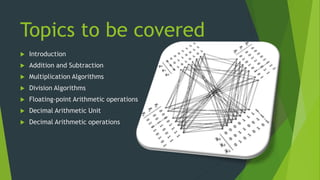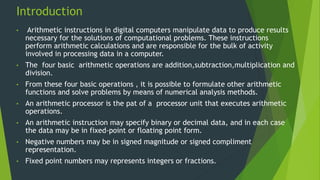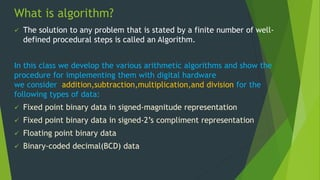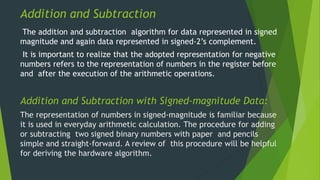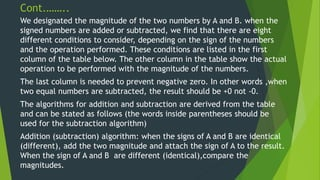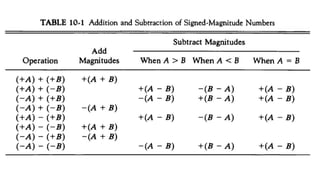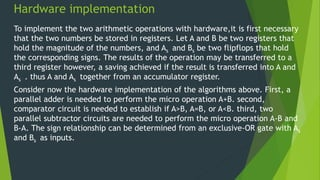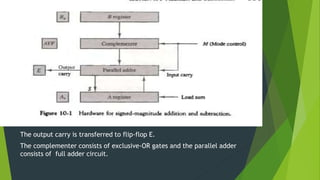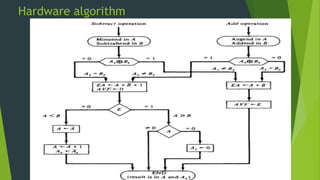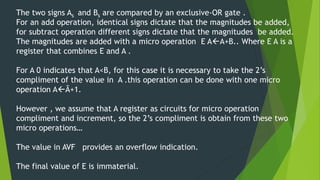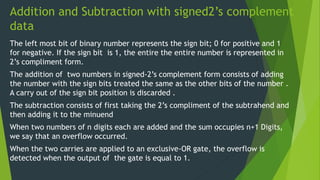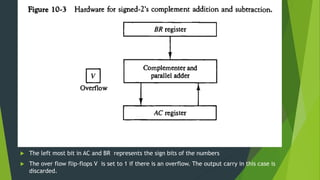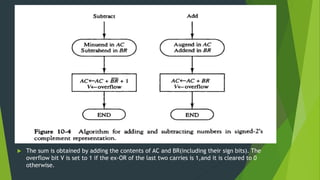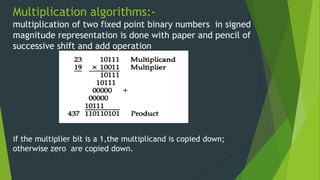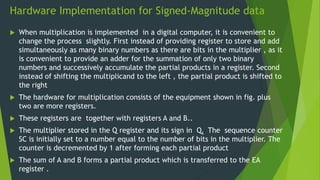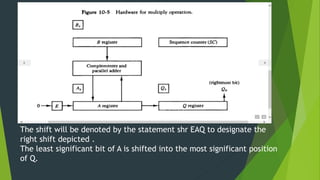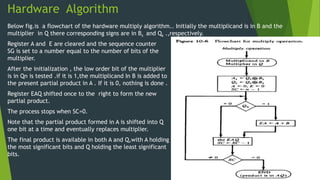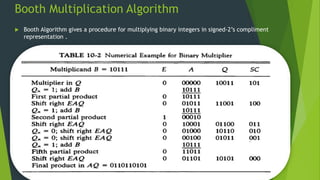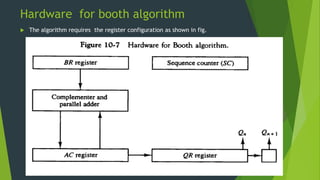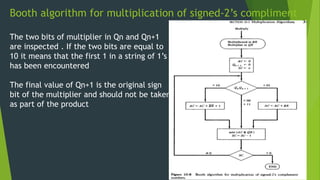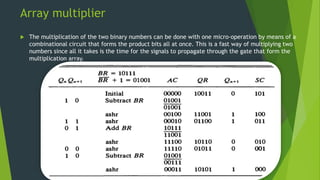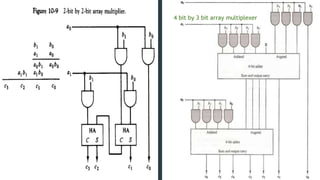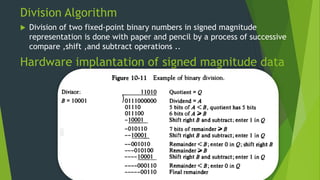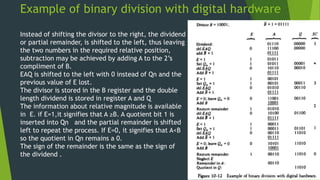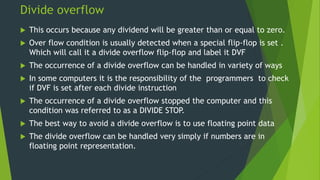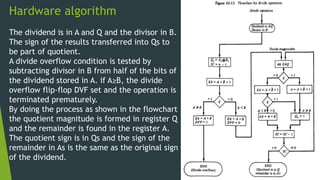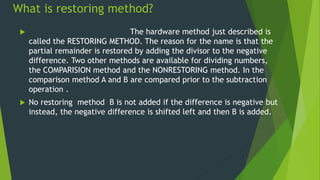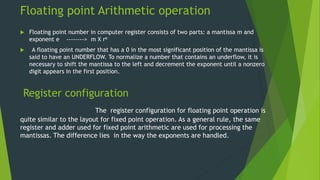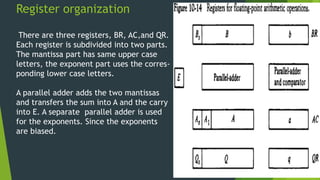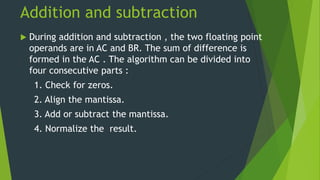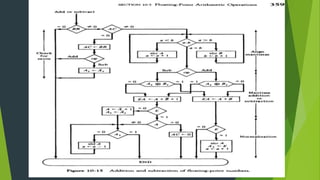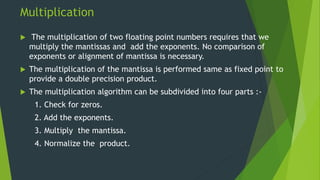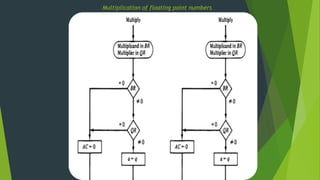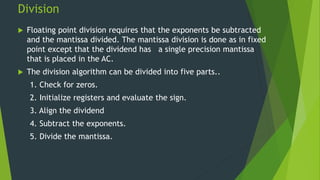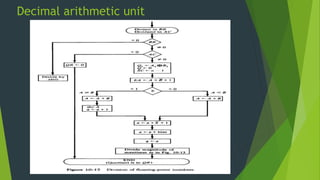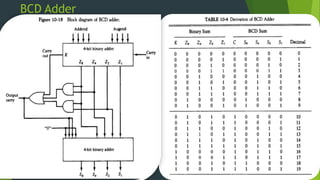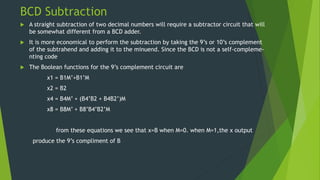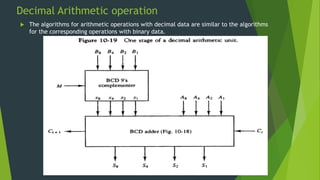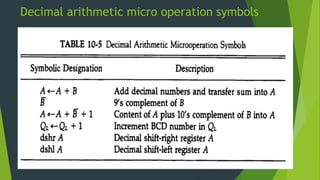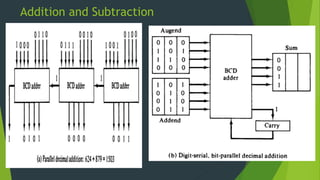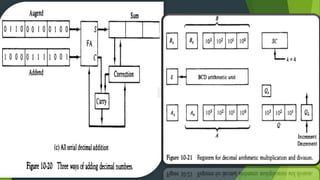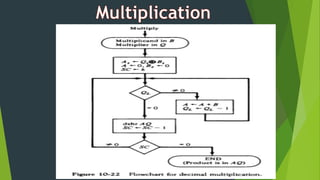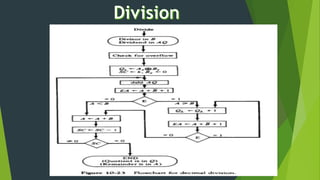1 de 46

### Computer arithmetic

• 2. Topics to be covered  Introduction  Addition and Subtraction  Multiplication Algorithms  Division Algorithms  Floating-point Arithmetic operations  Decimal Arithmetic Unit  Decimal Arithmetic operations
• 3. Introduction • Arithmetic instructions in digital computers manipulate data to produce results necessary for the solutions of computational problems. These instructions perform arithmetic calculations and are responsible for the bulk of activity involved in processing data in a computer. • The four basic arithmetic operations are addition,subtraction,multiplication and division. • From these four basic operations , it is possible to formulate other arithmetic functions and solve problems by means of numerical analysis methods. • An arithmetic processor is the pat of a processor unit that executes arithmetic operations. • An arithmetic instruction may specify binary or decimal data, and in each case the data may be in fixed-point or floating point form. • Negative numbers may be in signed magnitude or signed compliment representation. • Fixed point numbers may represents integers or fractions.
• 4. What is algorithm?  The solution to any problem that is stated by a finite number of well- defined procedural steps is called an Algorithm. In this class we develop the various arithmetic algorithms and show the procedure for implementing them with digital hardware we consider addition,subtraction,multiplication,and division for the following types of data:  Fixed point binary data in signed-magnitude representation  Fixed point binary data in signed-2’s compliment representation  Floating point binary data  Binary-coded decimal(BCD) data
• 5. Addition and Subtraction The addition and subtraction algorithm for data represented in signed magnitude and again data represented in signed-2’s complement. It is important to realize that the adopted representation for negative numbers refers to the representation of numbers in the register before and after the execution of the arithmetic operations. Addition and Subtraction with Signed-magnitude Data: The representation of numbers in signed-magnitude is familiar because it is used in everyday arithmetic calculation. The procedure for adding or subtracting two signed binary numbers with paper and pencils simple and straight-forward. A review of this procedure will be helpful for deriving the hardware algorithm.
• 6. Cont.…….. We designated the magnitude of the two numbers by A and B. when the signed numbers are added or subtracted, we find that there are eight different conditions to consider, depending on the sign of the numbers and the operation performed. These conditions are listed in the first column of the table below. The other column in the table show the actual operation to be performed with the magnitude of the numbers. The last column is needed to prevent negative zero. In other words ,when two equal numbers are subtracted, the result should be +0 not -0. The algorithms for addition and subtraction are derived from the table and can be stated as follows (the words inside parentheses should be used for the subtraction algorithm) Addition (subtraction) algorithm: when the signs of A and B are identical (different), add the two magnitude and attach the sign of A to the result. When the sign of A and B are different (identical),compare the magnitudes.
• 8. Hardware implementation To implement the two arithmetic operations with hardware,it is first necessary that the two numbers be stored in registers. Let A and B be two registers that hold the magnitude of the numbers, and As and Bs be two flipflops that hold the corresponding signs. The results of the operation may be transferred to a third register however, a saving achieved if the result is transferred into A and As . thus A and As together from an accumulator register. Consider now the hardware implementation of the algorithms above. First, a parallel adder is needed to perform the micro operation A+B. second, comparator circuit is needed to establish if A>B, A=B, or A<B. third, two parallel subtractor circuits are needed to perform the micro operation A-B and B-A. The sign relationship can be determined from an exclusive-OR gate with As and Bs as inputs.
• 9. The output carry is transferred to flip-flop E. The complementer consists of exclusive-OR gates and the parallel adder consists of full adder circuit.
• 10. Hardware algorithm
• 11. The two signs As and Bs are compared by an exclusive-OR gate . For an add operation, identical signs dictate that the magnitudes be added, for subtract operation different signs dictate that the magnitudes be added. The magnitudes are added with a micro operation E AA+B.. Where E A is a register that combines E and A . For A 0 indicates that A<B, for this case it is necessary to take the 2’s compliment of the value in A .this operation can be done with one micro operation AĀ+1. However , we assume that A register as circuits for micro operation compliment and increment, so the 2’s compliment is obtain from these two micro operations… The value in AVF provides an overflow indication. The final value of E is immaterial.
• 12. Addition and Subtraction with signed2’s complement data The left most bit of binary number represents the sign bit; 0 for positive and 1 for negative. If the sign bit is 1, the entire the entire number is represented in 2’s compliment form. The addition of two numbers in signed-2’s complement form consists of adding the number with the sign bits treated the same as the other bits of the number . A carry out of the sign bit position is discarded . The subtraction consists of first taking the 2’s compliment of the subtrahend and then adding it to the minuend When two numbers of n digits each are added and the sum occupies n+1 Digits, we say that an overflow occurred. When the two carries are applied to an exclusive-OR gate, the overflow is detected when the output of the gate is equal to 1.
• 13.  The left most bit in AC and BR represents the sign bits of the numbers  The over flow flip-flops V is set to 1 if there is an overflow. The output carry in this case is discarded.
• 14.  The sum is obtained by adding the contents of AC and BR(including their sign bits). The overflow bit V is set to 1 if the ex-OR of the last two carries is 1,and it is cleared to 0 otherwise.
• 15. Multiplication algorithms:- multiplication of two fixed point binary numbers in signed magnitude representation is done with paper and pencil of successive shift and add operation if the multiplier bit is a 1,the multiplicand is copied down; otherwise zero are copied down.
• 16. Hardware Implementation for Signed-Magnitude data  When multiplication is implemented in a digital computer, it is convenient to change the process slightly. First instead of providing register to store and add simultaneously as many binary numbers as there are bits in the multiplier , as it is convenient to provide an adder for the summation of only two binary numbers and successively accumulate the partial products in a register. Second instead of shifting the multiplicand to the left , the partial product is shifted to the right  The hardware for multiplication consists of the equipment shown in fig. plus two are more registers.  These registers are together with registers A and B..  The multiplier stored in the Q register and its sign in Qs The sequence counter SC is initially set to a number equal to the number of bits in the multiplier. The counter is decremented by 1 after forming each partial product  The sum of A and B forms a partial product which is transferred to the EA register .
• 17. The shift will be denoted by the statement shr EAQ to designate the right shift depicted . The least significant bit of A is shifted into the most significant position of Q.
• 18. Hardware Algorithm Below fig.is a flowchart of the hardware multiply algorithm.. Initially the multiplicand is in B and the multiplier in Q there corresponding signs are in Bs and Qs .,respectively. Register A and E are cleared and the sequence counter SG is set to a number equal to the number of bits of the multiplier. After the initialization , the low order bit of the multiplier is in Qn is tested .if it is 1,the multiplicand In B is added to the present partial product in A . If it is 0, nothing is done . Register EAQ shifted once to the right to form the new partial product. The process stops when SC=0. Note that the partial product formed in A is shifted into Q one bit at a time and eventually replaces multiplier. The final product is available in both A and Q,with A holding the most significant bits and Q holding the least significant bits.
• 19. Booth Multiplication Algorithm  Booth Algorithm gives a procedure for multiplying binary integers in signed-2’s compliment representation .
• 20. Hardware for booth algorithm  The algorithm requires the register configuration as shown in fig.
• 21. Booth algorithm for multiplication of signed-2’s compliment The two bits of multiplier in Qn and Qn+1 are inspected . If the two bits are equal to 10 it means that the first 1 in a string of 1’s has been encountered The final value of Qn+1 is the original sign bit of the multiplier and should not be taken as part of the product
• 22. Array multiplier  The multiplication of the two binary numbers can be done with one micro-operation by means of a combinational circuit that forms the product bits all at once. This is a fast way of multiplying two numbers since all it takes is the time for the signals to propagate through the gate that form the multiplication array.
• 23. 4 bit by 3 bit array multiplexer
• 24. Division Algorithm  Division of two fixed-point binary numbers in signed magnitude representation is done with paper and pencil by a process of successive compare ,shift ,and subtract operations .. Hardware implantation of signed magnitude data
• 25. Example of binary division with digital hardware Instead of shifting the divisor to the right, the dividend or partial remainder, is shifted to the left, thus leaving the two numbers in the required relative position, subtraction may be achieved by adding A to the 2’s compliment of B. EAQ is shifted to the left with 0 instead of Qn and the previous value of E lost. The divisor is stored in the B register and the double length dividend is stored in register A and Q The information about relative magnitude is available in E. if E=1,it signifies that A ≥B. A quotient bit 1 is inserted into Qn and the partial remainder is shifted left to repeat the process. If E=0, it signifies that A<B so the quotient in Qn remains a 0. The sign of the remainder is the same as the sign of the dividend .
• 26. Divide overflow  This occurs because any dividend will be greater than or equal to zero.  Over flow condition is usually detected when a special flip-flop is set . Which will call it a divide overflow flip-flop and label it DVF  The occurrence of a divide overflow can be handled in variety of ways  In some computers it is the responsibility of the programmers to check if DVF is set after each divide instruction  The occurrence of a divide overflow stopped the computer and this condition was referred to as a DIVIDE STOP.  The best way to avoid a divide overflow is to use floating point data  The divide overflow can be handled very simply if numbers are in floating point representation.
• 27. Hardware algorithm The dividend is in A and Q and the divisor in B. The sign of the results transferred into Qs to be part of quotient. A divide overflow condition is tested by subtracting divisor in B from half of the bits of the dividend stored in A. if A≥B, the divide overflow flip-flop DVF set and the operation is terminated prematurely. By doing the process as shown in the flowchart the quotient magnitude is formed in register Q and the remainder is found in the register A. The quotient sign is in Qs and the sign of the remainder in As is the same as the original sign of the dividend.
• 28. What is restoring method?  The hardware method just described is called the RESTORING METHOD. The reason for the name is that the partial remainder is restored by adding the divisor to the negative difference. Two other methods are available for dividing numbers, the COMPARISION method and the NONRESTORING method. In the comparison method A and B are compared prior to the subtraction operation .  No restoring method B is not added if the difference is negative but instead, the negative difference is shifted left and then B is added.
• 29. Floating point Arithmetic operation  Floating point number in computer register consists of two parts: a mantissa m and exponent e --------> m X re  A floating point number that has a 0 in the most significant position of the mantissa is said to have an UNDERFLOW. To normalize a number that contains an underflow, it is necessary to shift the mantissa to the left and decrement the exponent until a nonzero digit appears in the first position. Register configuration The register configuration for floating point operation is quite similar to the layout for fixed point operation. As a general rule, the same register and adder used for fixed point arithmetic are used for processing the mantissas. The difference lies in the way the exponents are handled.
• 30. Register organization There are three registers, BR, AC,and QR. Each register is subdivided into two parts. The mantissa part has same upper case letters, the exponent part uses the corres- ponding lower case letters. A parallel adder adds the two mantissas and transfers the sum into A and the carry into E. A separate parallel adder is used for the exponents. Since the exponents are biased.
• 31. Addition and subtraction  During addition and subtraction , the two floating point operands are in AC and BR. The sum of difference is formed in the AC . The algorithm can be divided into four consecutive parts : 1. Check for zeros. 2. Align the mantissa. 3. Add or subtract the mantissa. 4. Normalize the result.
• 33. Multiplication  The multiplication of two floating point numbers requires that we multiply the mantissas and add the exponents. No comparison of exponents or alignment of mantissa is necessary.  The multiplication of the mantissa is performed same as fixed point to provide a double precision product.  The multiplication algorithm can be subdivided into four parts :- 1. Check for zeros. 2. Add the exponents. 3. Multiply the mantissa. 4. Normalize the product.
• 34. Multiplication of floating point numbers
• 35. Division  Floating point division requires that the exponents be subtracted and the mantissa divided. The mantissa division is done as in fixed point except that the dividend has a single precision mantissa that is placed in the AC.  The division algorithm can be divided into five parts.. 1. Check for zeros. 2. Initialize registers and evaluate the sign. 3. Align the dividend 4. Subtract the exponents. 5. Divide the mantissa.
• 36. Decimal arithmetic unit